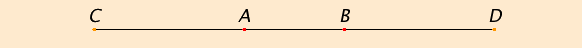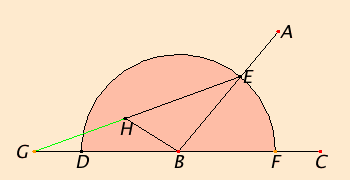# Postulate 2

To produce a finite straight line continuously in a straight line.

## GuideHere we have the second ability of a straightedge, namely, to extend a given line AB to CD. This postulate does not say how far a line can be extended. Sometimes it is used so that the extension equals some other line. Other times it is extended arbitrarily far.

As with the first postulate, it is implicitly assumed in the books on plane geometry that when a line is extended, it remains in the plane of discussion. The first proposition on solid geometry, proposition XI.1, claims that line can’t be only partly in a plane. The central step in the proof of that proposition is to show that a line cannot be extended in two ways, that is, there is only one continuation of a line. The proof is hardly convincing. Rather, this postulate should include a clause to that effect.

#### Neusis: fitting a line into a diagram

Other uses of a straightedge can be imagined. For instance, it might be marked at two points on it, then fit into a diagram so that the two points fall on two lines, perhaps curved. This operation is an example of “neusis” or “verging” where lines are adjusted to fit the diagram. For instance, Archimedes, who lived in the century after Euclid, used neusis in several constructions in his work On Spirals. In the Book of Lemmas, attributed by Thabit ibn-Qurra to Archimedes, neusis is used to trisect an angle.
 Suppose the angle ABC is to be trisected. Draw a circle DEF with center B and any radius. Extend CB through D and beyond. Fit in a line GHE passing through E and a point G on the line CB extended so that a segment (colored green) equal to the radius BD of the circle starts at G and ends at a point H on the circle. (You’ll have to move G around until H lands on the circle.) Draw BH. With the help of Euclid’s propositions here in Book I, we can show that angle EGC is one-third of angle ABC. Since the lines GH, HB, and BE are equal, therefore the triangles GHB and HBE are isosceles. Therefore, by I.5, angle HGB equals HBG, and angle BHE equals angle BEH.By I.32, the exterior angle BHE of triangle GHB equals the sum of the equal angles HGB and HBG, therefore angle BHE is double angle HGB. And angle BEH equals BHE, so it is also double angle HGB.

Again by I.32, the exterior angle ABC of triangle BEG is the sum of angles HGB and BEH. But angle BEH is double angle HGB, therefore angle ABC is triple angle HGB.

Therefore, angle GHB is one-third of angle ABC. Q.E.D.

The ancient Greek geometers believed that angle trisection required tools beyond those given in Euclid’s postulates. They were right, but it wasn’t proved until Wantzel in 1837 proved that a 60°-angle cannot be so trisected using only Euclidean tools.

Euclid has no postulate for neusis constructions, and since neusis constructions can trisect angles, we conclude from Wantzel’s theorem that another postulate is required to justify neusis constructions.